# Right Circular Cone Basics

Right Circular Cone Basics
Go back to  'Solid Shapes'

Try imagining a triangle rotating.

The 3D shape so formed will be a cone!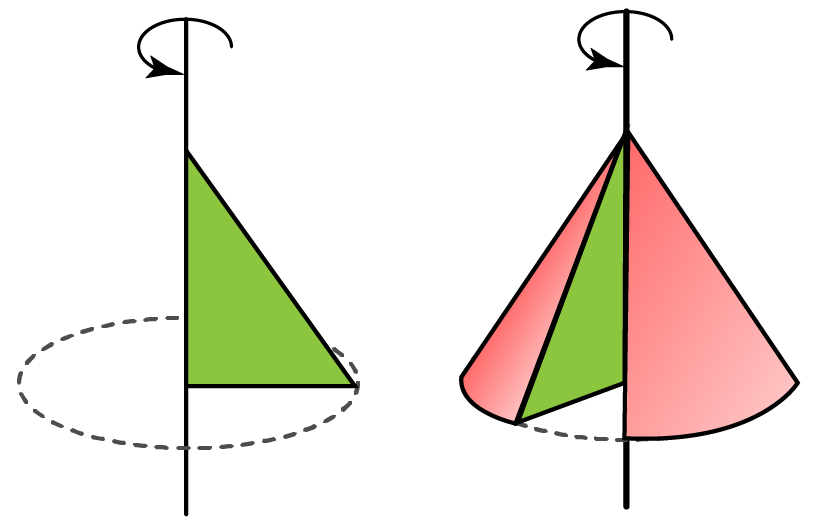Any triangle will form a cone when it is rotated, taking one of its two short sides as the axis of rotation.

A cone is a 3D geometric figure that has a flat circular surface and a curved surface that meets at a point toward the top.

The point formed at the end of the cone is called the apex or vertex, whereas the flat surface is called the base.

Other parts of the cone are shown in the below diagram: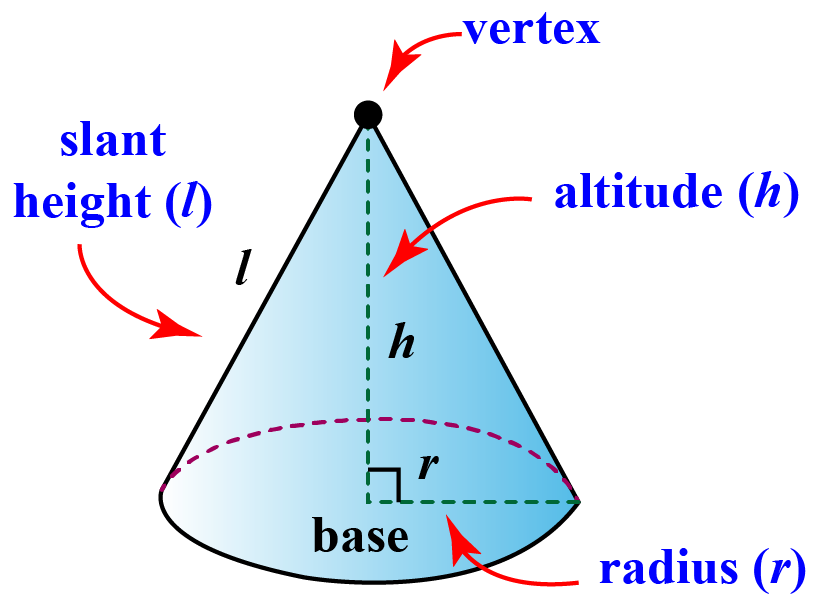Let's learn more amazing facts and properties related to this interesting  3D-shape as we scroll down.

## Lesson Plan

 1 What Is a Right Circular Cone? 2 Challenging Questions on Right Circular Cone 3 Important Notes on Right Circular Cone 4 Solved Examples on Right Circular Cone 5 Interactive Questions on Right Circular Cone

## What Is a Right Circular Cone?

A right circular cone is one whose axis is perpendicular to the plane of the base.

A right circular cone is generated by a revolving a right triangle about one of its legs.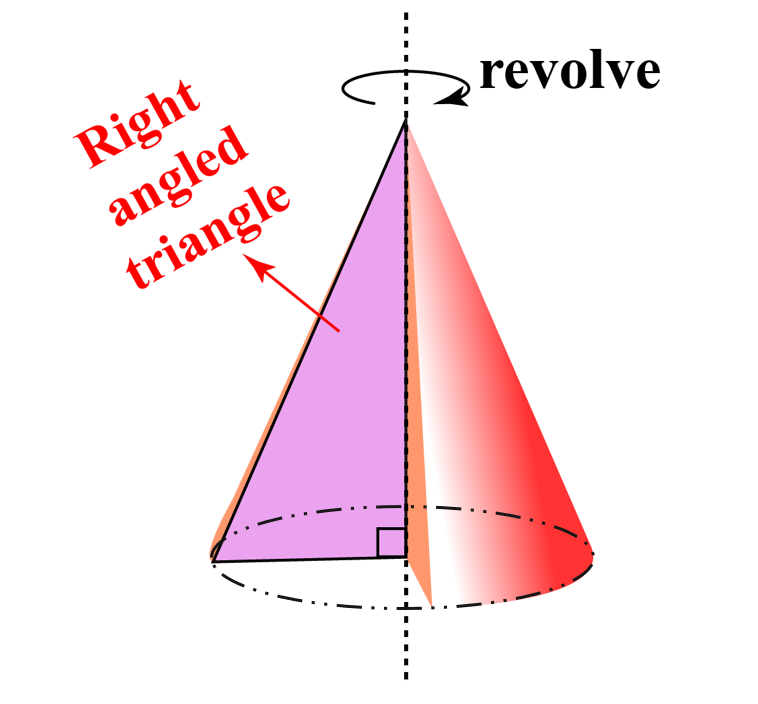## Right Circular Cone vs Oblique Cone

A right circular cone or regular cone's axis is perpendicular to its base, whereas the oblique cone appears to be tilted and its axis is not perpendicular to the base.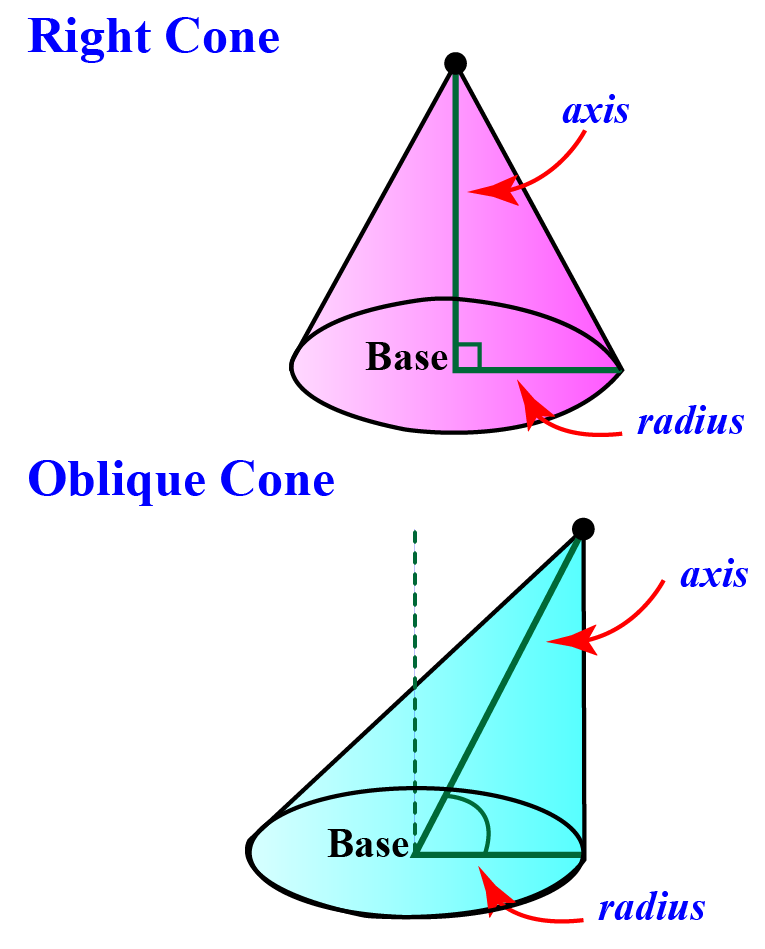Another way to check if a cone is a right circular cone is to check its cross-section in a horizontal plane.

A right circular cone will give a circular cross-section, whereas an oblique cone will give an oval cross-section.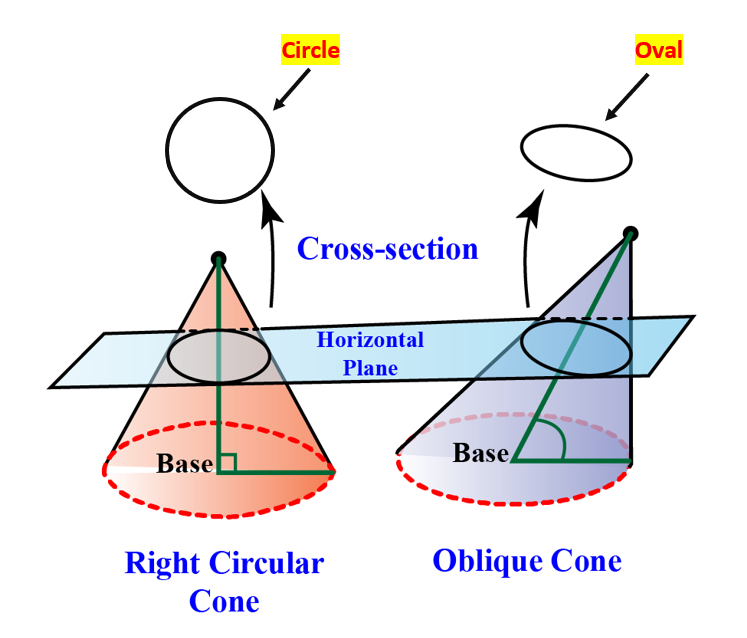## Surface Areas of a Cone (Curved and Total)

To learn about the surface area of a cone, we cut and open up the cone.

The curved surface forms a sector with radius $$s$$, as shown below.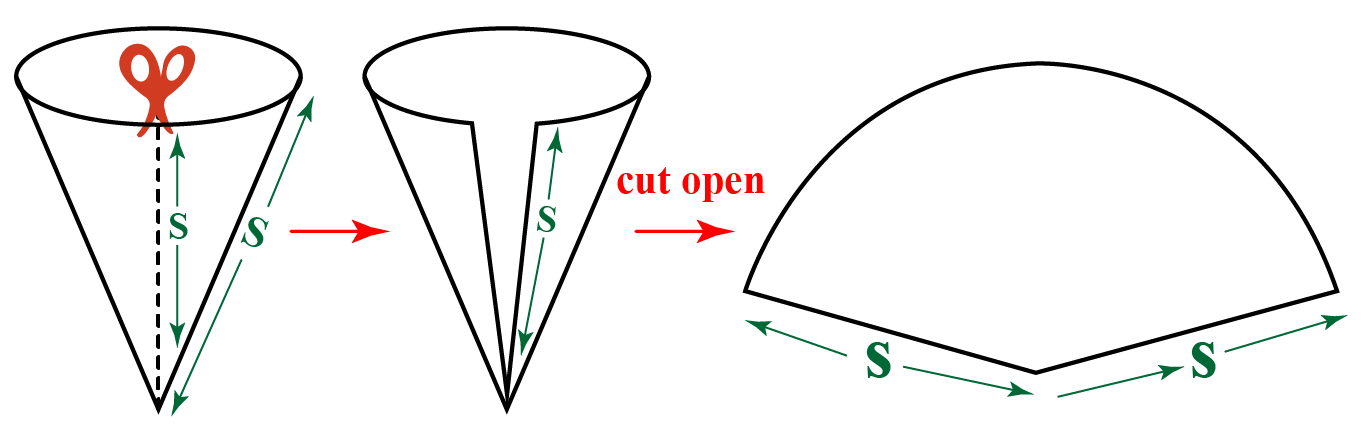The curved surface area of a cone = Area of the sector with radius length equal to the slant height i.e., $$s$$

 Curved Surface Area of a Cone = $$\pi rs$$ = $$\pi r\sqrt{r^{2}+h^{2}}$$

The total surface area of a cone = Area of circular base + Curved surface area of a cone (sector's area)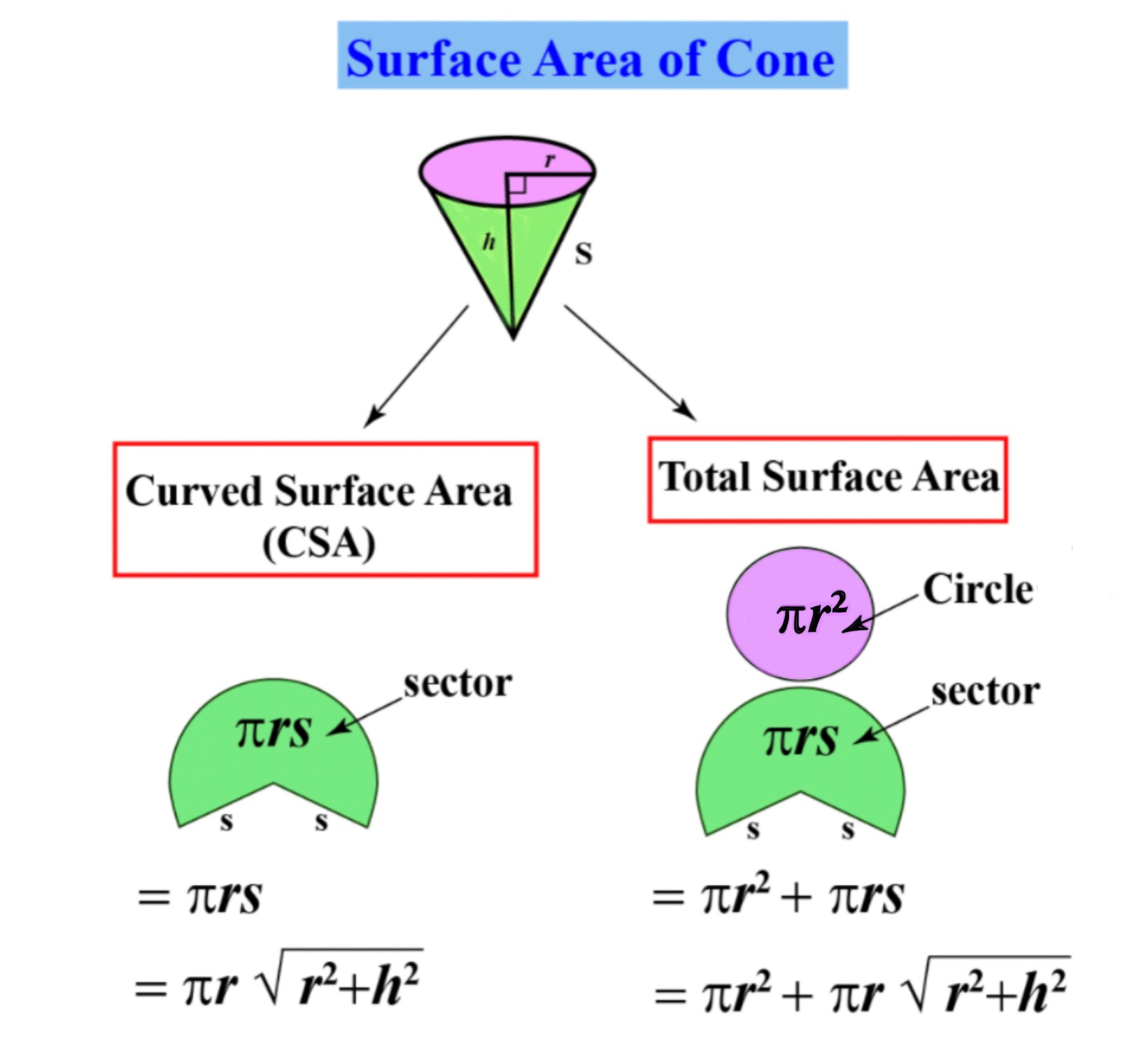Total Surface Area of a Cone = $$\pi r^{2} + \pi rs$$
 Total Surface Area of a Cone = $$\pi r^{2} + \pi r\sqrt{r^{2}+h^{2}}$$

Curved surface area is also known as the lateral surface area.

The surface area is measured in terms of square units.

## Volume of a Right Circular Cone/ Volume of a Cone

The volume of a cone that has a circular base with radius $$r$$ and height $$h$$ will be equal to one-third of the product of the area of the base and its height.

Therefore,

\begin{align*}\text{Volume of a Cone} (V) &= \dfrac{1}{3} \times \text{Area of Circular Base} \times \text{Height of the Cone}\\ V &= \dfrac{1}{3} \times \pi r^{2} \times h\end{align*}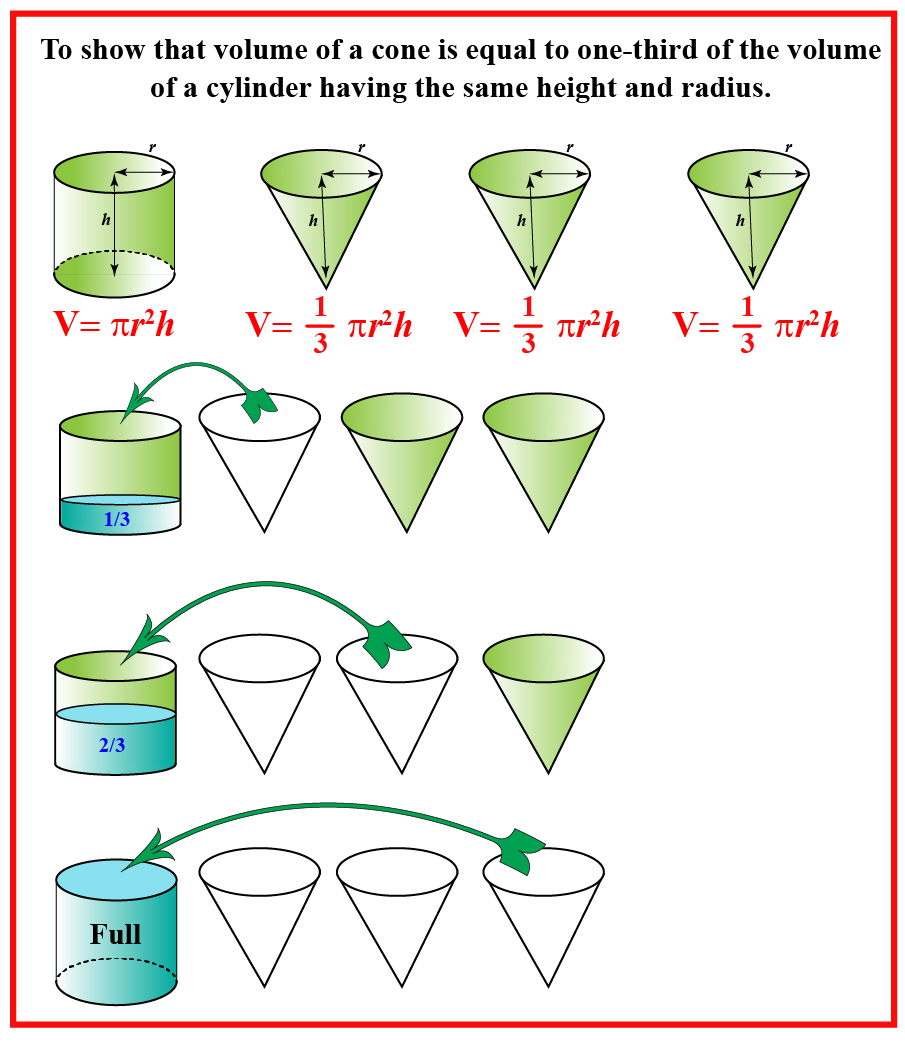$$\text{Volume of a Cone} = \dfrac{1}{3} \times \pi r^{2}h$$

## Right Circular Cone Calculator

Here's a calculator to calculate the surface area and volume of a cone.

Enter the values for the base and height and it will do the desired calculations for you.Challenging Questions
• Two children planned to dig a pit in the ground in the shape of a cone.
They dug out $$48\pi$$ cubic units of mud to form the pit.
If the depth of the pit was 9 units, find the radius of the pit.
• Sam knows that the ratio of the volume of a cone and the volume of a cylinder with the same height and radius is 1:3.
How will you help him prove that?

## Properties of a Right Circular Cone

• It has a circular base. The axis is a line that joins the vertex to the center of the base.
• The slant height of the cone is measured from the vertex to the edge of the circular base. It is denoted by $$l$$ or $$s.$$
• The altitude or height of a right cone coincides with the axis of the cone and is represented by $$h.$$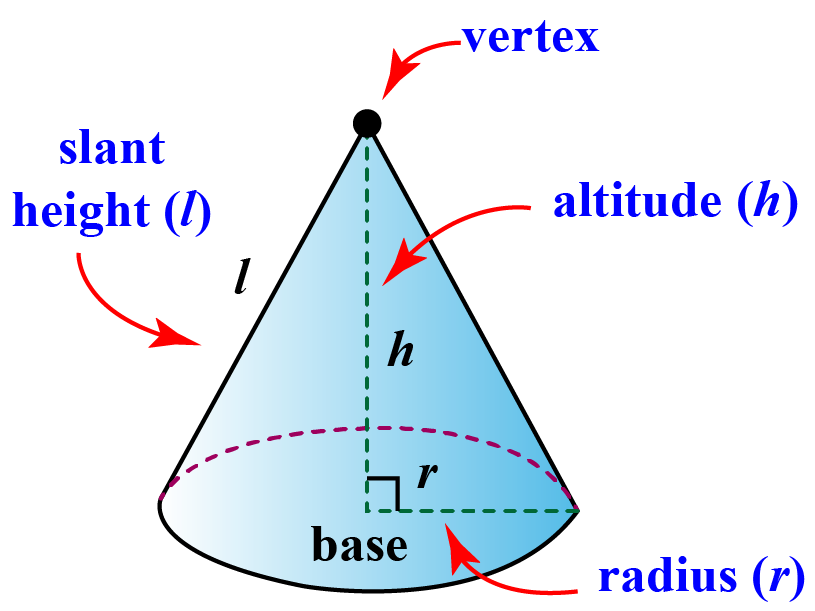• If a right triangle is rotated with the perpendicular side as the axis of rotation, a right circular cone is constructed. The surface area generated by the hypotenuse of the triangle is the curved surface or the lateral surface area.
• Any horizontal section of the right circular cone parallel to the base produces the cross-section of a circle.
• A plane that passes through the vertex and any two points of the base of a right circular cone generates an isosceles triangle as given below: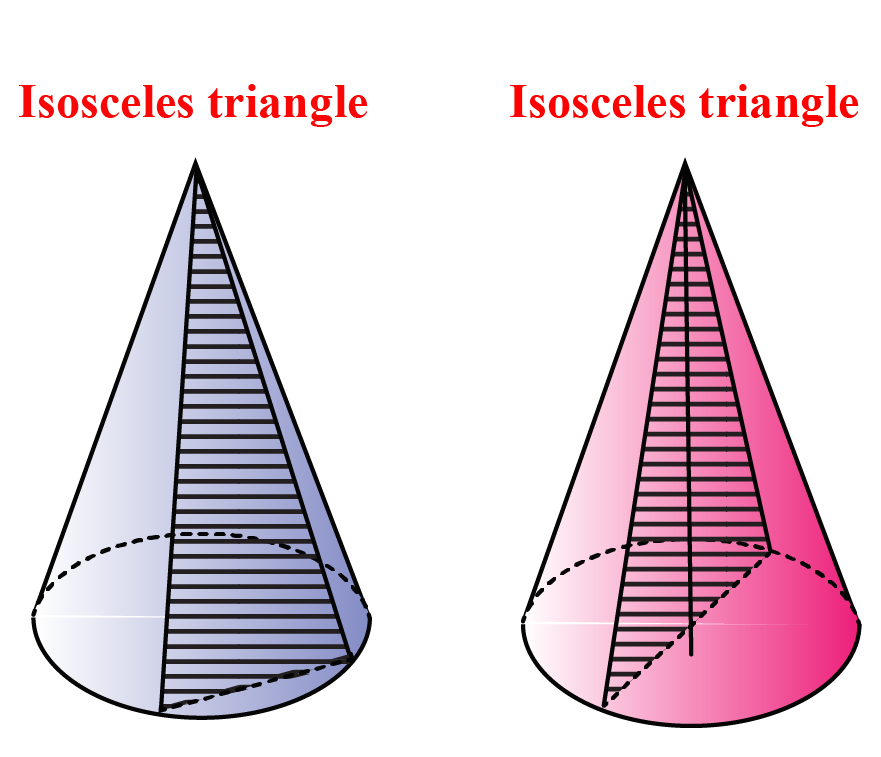Mensuration and Solids
grade 10 | Questions Set 2
Mensuration and Solids
Mensuration and Solids
grade 10 | Questions Set 1
Mensuration and Solids